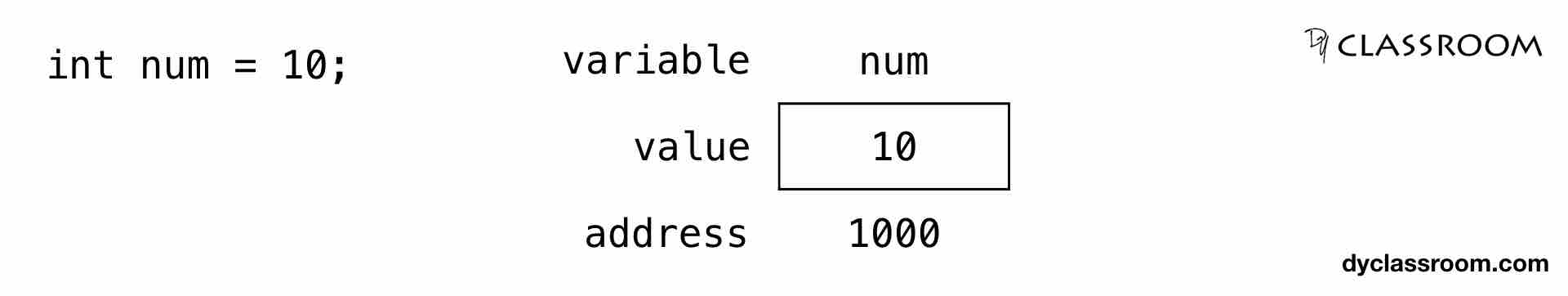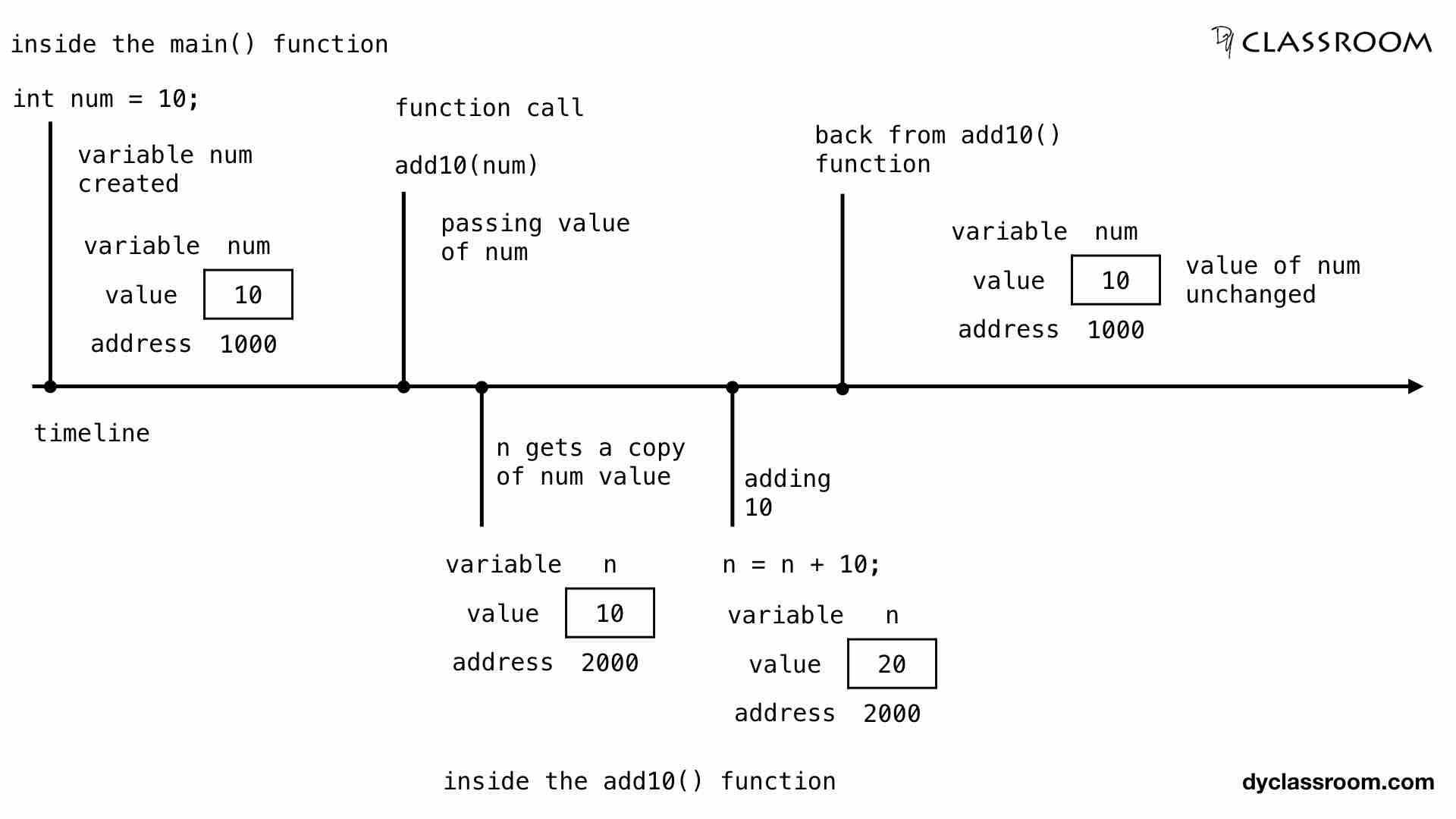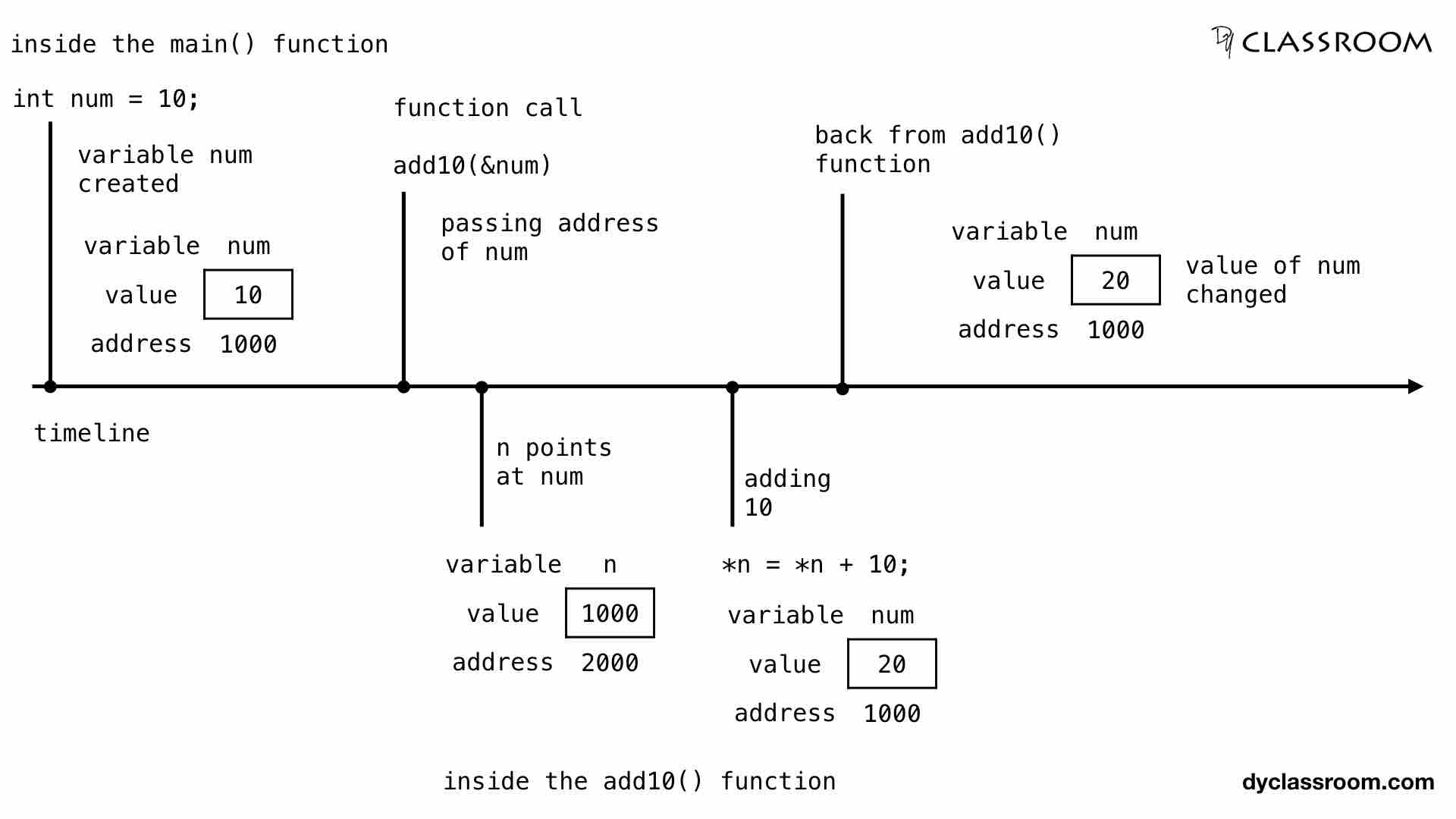# C - Pointers and Functions - Call by Value and Call by Reference

C ProgrammingIn this tutorial we will learn to use pointers with functions in C programming language.

We covered functions in the previous tutorials. Feel free to check that out.

## Create a variable

We will start by creating an integer variable `num`.

``````#include <stdio.h>

int main(void) {

// integer variable
int num = 10;

return 0;
}
``````

We can represent this in memory as follows.## Passing value to function

There are two ways we can pass value to a function.

## Call by value

In this method we pass a copy of the variable and not the actual variable to the called function. So, any change made to the copy of the variable in the called function is not reflected back to the original variable.

In the following example we have the `num` integer variable. We are calling the `add10()` function and passing the `num` variable as an argument to the function.

``````#include <stdio.h>

// function declaration

int main(void) {

// integer variable
int num = 10;

// print value of num
printf("Value of num before function call: %d\n", num);

// pass by value

// print value of num
printf("Value of num after function call: %d\n", num);

return 0;
}

// function definition
n = n + 10;
}
``````

Output:

``````Value of num before function call: 10
Value of num after function call: 10
``````

We can see that the value of `num` is not changed after the function call. This is because when we pass the `num` variable by value as argument to the function `add10()` then, inside the `add10()` function we work with a copy `n` and not with the actual variable `num`.

In the following image we can see that `num` variable is saved in memory location 1000 and the parameter variable `n` of the function `add10()` gets stored at memory location 2000.When `num` is passed to the function, the value of num i.e., 10 is saved in the location 2000.

And then we add 10 to `n` inside the `add10()` function. So, the new value at location 2000 becomes 20. But the value at location 1000 where the `num` variable is stored still remains 10. Hence we get the above result were `num` remains unchanged.

If we want to work with the variable `num` then we have to take help of pointer and pass `num` by reference.

## Function taking pointers as argument

Following is the declaration syntax of a function to take pointers as argument.

``````returnType functionName(dataType *ptrVar);
``````

Where `returnType` is the return type of the function. So, if the function will return no value then set it to `void`.

`functionName` is the name of the function.

`dataType` represents the data type of the pointer variable `ptrVar`.

## Call by reference

In this case we pass the address of the variable `num` as argument to the `add10()` function.

``````#include <stdio.h>

// function declaration

int main(void) {

// integer variable
int num = 10;

// print value of num
printf("Value of num before function call: %d\n", num);

// pass by reference

// print value of num
printf("Value of num after function call: %d\n", num);

return 0;
}

// function definition
*n = *n + 10;
}
``````

Output:

``````Value of num before function call: 10
Value of num after function call: 20
``````

We can see that in the above output the value of num gets changed.

In the following image we can see that the `num` variable is stored at memory location 1000.When we are calling the `add10()` function we are passing the address of `num` to the function as argument.

So, the function parameter variable `n` which is allocated the memory location 2000, stores the address of the `num` variable i.e., 1000. So, n is pointing at num.

Inside the `add10()` function we are adding 10 using the following code.

``````*n = *n + 10;
``````

Where, `*n` means the value at the address stored in the pointer variable `n`.

So, address stored inside pointer variable `n` is 1000 which points at the variable `num`. So, `*n` gives us 10 i.e., value of `num`.

Since we are updating the value at the location 1000 from inside the `add10()` function so, when we return back from the function call we get the updated value of `num` variable.